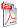Issue archive

https://doi.org/10.15255/KUI.2003.022
Published: Kem. Ind. 53 (3) (2004) 109–115
Paper reference number: KUI-22/2003
Paper type: Original scientific paper
Download paper:PDFEvaluation of the Characteristic Drying Process Parameters

A. Sander and A. Glasnović

##### Abstract

Mathematical modelling of drying is a very difficult task due to the changing states of the moist product during drying and many different dryer types. To date, no uniform design and mathematical model for dryers exist. The most used models are so called thin-layer models (Table 1). In the world, there are two major opinions that exist. One group of authors claims that there are purely empirical models, and no one expects to find physical meaning of the model parameters. On the other hand, the other group of authors states quite the opposite. The objective of this work is to point out the physical meaning of drying model parameter and to find out how the heating intensity and granulometric properties of material influence the drying kinetics and model parameters for various types of materials. Drying data were correlated with new modification of Page's thin-layer model. The modification was made in order to define physical meaning of one model parameter. Experiments were carried out on a laboratory scale dryer. Microwave drying of four different fractions of pharmaceutical powder were performed at various microwave power levels (Table 2, Figure 1, Figure 2). Experimental drying rate curves show the existence of two falling rate periods (Figure 5). During the first linear falling rate period, the controling mechanism of internal moisture migration is capillary flow. Exponential form of the second falling rate period speaks on behalf of diffusion. Obtained results show that applied mathematical model describes drying kinetics very well (Figure 4). It turned out that one model parameter directly define the moment when diffusion as the governing moisture removal mechanism starts. In other words, this parameter, tk, is the time wheh second, if it exists, falling rate period starts. The other model parameter, n, does not depend of microwave power level, so it was supposed that its value depends on the way that heat is supplied to the material (i.e. drying method). At higher drying rates and smaller specific surface area, estimated values of parameter tk were lower, so diffusion takes place earlier (Figure 6). For the second falling rate period, effective moisture diffusion coefficients are calculated numerically from experimental data and related to drying conditions and material properites. Values of the effective diffusion coefficient increase with increasing microwave heating power and decreasing specific surface area (Figure 7, Figure 9). The influence of the specific surface area can be explained with stronger attraction forces between smaller particles (Figure 2). This leads to a higher resistance to internal mass transfer, and the drying process is slower (Figure 8).

##### Keywords

microwave drying, mathematical model, effective diffusion coefficient